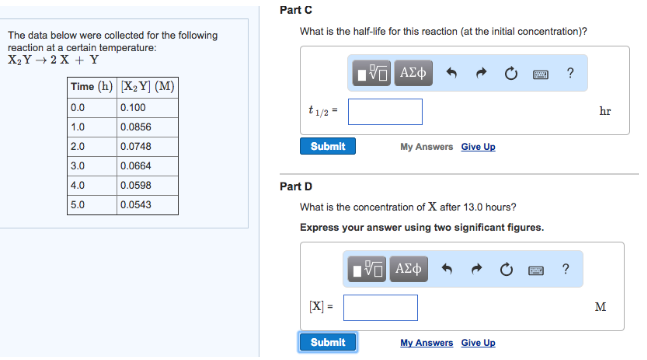# Problem: The data below were collected for the following reaction at a certain temperature: X2Y → 2X + Y What is the half-life for this reaction (at the initial concentration)?What is the concentration of X after 13.0 hours? Express your answer using two significant figures.

###### FREE Expert Solution
97% (160 ratings)###### Problem Details

The data below were collected for the following reaction at a certain temperature:

X2Y → 2X + Y

What is the half-life for this reaction (at the initial concentration)?

What is the concentration of X after 13.0 hours? Express your answer using two significant figures.